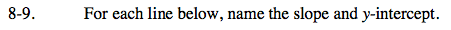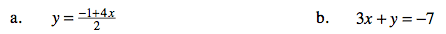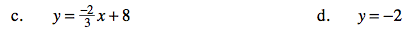### Home > CAAC > Chapter 8 > Lesson 8.1.1 > Problem8-9

8-9.This equation, rewritten, is the same as saying:

$y=\frac{4}{2}x-\frac{1}{2}$

Knowing this information, can you identify the y-intercept and the slope?

$\text{Slope}=\frac{4}{2}=2 \ \ \ \ \ \ \ y\text{-intercept}=-\frac{1}{2}$

Rewrite the equation in y = mx + b form, then identify the slope and the y-intercept.The equation is already in y = mx + b form, so identify the slope and y-intercept in the equation.

Remember that y = −2 is a horizontal line.

Because it is horizontal, the line has zero slope and the y-intercept is given right in the equation, −2.